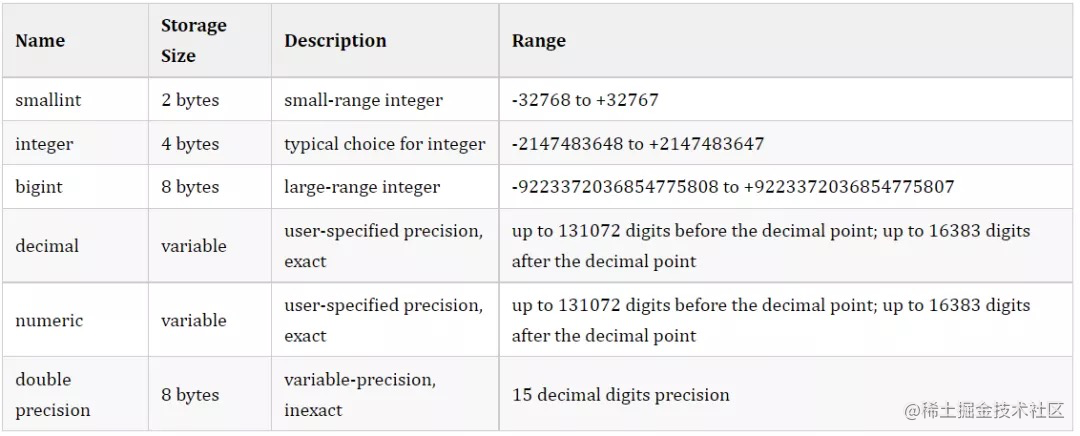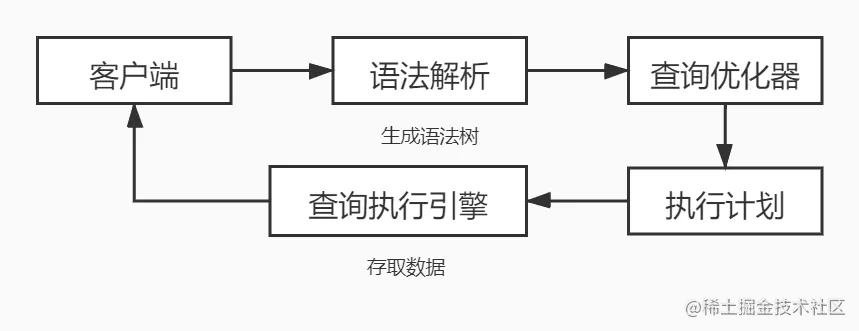# 为金融场景而生的数据类型：Numeric

c 源码 ：github.com/postgres/po…

# Numeric 语法简介

NUMERIC(precision, scale)

• precision：numeric 中全部数字个数的总和

• scale：小数点后面的数字个数

1. 所有的整数都可以看成 scale 为 0 的 numeric；

2. precision 必须为正数，scale 可以为 0 或者正数；

3. numeric(precision) 语法，默认的 scale 是 0；

4. 语法中不带任何参数，则任意 precision 和 scale 的值都可以被存储，只要不超过 precision 的最大值；

5. 只要 numeric 中声明了 scale，则输入的值都要强制的去匹配这个 scale（即进行 round 操作，round 为四舍五入）；

6. 如果输入的 scale 数值溢出，则报错。# Numeric 特殊值

``````	UPDATE table SET x = 'NaN'

# SQL 中 Numeric 数据流向``````	CREATE TABLE test (
name VARCHAR(100) NOT NULL,
price NUMERIC(5,2)
);

INSERT INTO test (name, price)
VALUES ('Phone',500.215),
('Tablet',500.214);

# Numeric 磁盘存储结构解析

``````	struct NumericLong
{
uint16          n_sign_dscale;  /* Sign + display scale */
int16           n_weight;               /* Weight of 1st digit  */
NumericDigit n_data[FLEXIBLE_ARRAY_MEMBER]; /* Digits */
};

struct NumericShort
{
uint16          n_header;               /* Sign + display scale + weight */
NumericDigit n_data[FLEXIBLE_ARRAY_MEMBER]; /* Digits */
};

union NumericChoice
{
struct NumericLong n_long;      /* Long form (4-byte header) */
struct NumericShort n_short;    /* Short form (2-byte header) */
};

struct NumericData
{
int32           vl_len_;                /* varlena header (do not touch directly!) */
union NumericChoice choice; /* choice of format */
};

## 结构体 NumericLong

``````	struct NumericLong
{
uint16          n_sign_dscale;  /* Sign + display scale */
int16           n_weight;               /* Weight of 1st digit  */
NumericDigit n_data[FLEXIBLE_ARRAY_MEMBER]; /* Digits */
};

**uint16 n_sign_dscale **：第一个字节中高两位 bit 用于保存正负号。 若为 0x0000：则符号位正

int16 n_weight ：保存权值。这里要解释下权值在这里的含义。在这里 numeric 是用一组 int16 数组表示的，每一个元素用 int16 表示 4 位数字，也就是最大保存 9999。那么基数 base 值就是 10000。这里的权值的 base 值就是 10000（10 进制的权值 base 值是 10，二进制是 2）。**NumericDigit n_data[FLEXIBLE_ARRAY_MEMBER] **：动态数组（也有叫柔性数组的，在这里统一称动态数组吧），是 C99 之后添加的一个特性。这个特性是在这个结构体中，动态数组并不占用任何空间，其长度由 NumericData 中的 vl_len_ 决定。

## 结构体 NumericShort

``````	struct NumericShort
{
uint16          n_header;               /* Sign + display scale + weight */
NumericDigit n_data[FLEXIBLE_ARRAY_MEMBER]; /* Digits */
};

NumericDigit n_data[FLEXIBLE_ARRAY_MEMBER] ：参考上文柔性数组描述。

## 联合体 NumericChoice

``````	union NumericChoice
{
struct NumericLong n_long;      /* Long form (4-byte header) */
struct NumericShort n_short;    /* Short form (2-byte header) */
};

0x8000：则采用 NumericShort 存储格式

0xC000：则为 NaN

## 结构体 NumericData

``````	struct NumericData
{
int32           vl_len_;                /* varlena header (do not touch directly!) */
union NumericChoice choice; /* choice of format */
};

int32 vl_len_ ：用来保存动态数组的长度，这个数组是 NumericLong 或者 NumericShort 结构体中定义的动态数组。

## Numeric 内存计算结构解析

``````	typedef struct NumericVar
{
int                     ndigits;                /* # of digits in digits[] - can be 0! */
int                     weight;                 /* weight of first digit */
int                     sign;                   /* NUMERIC_POS, NUMERIC_NEG, or NUMERIC_NAN */
int                     dscale;                 /* display scale */
NumericDigit *buf;                      /* start of palloc'd space for digits[] */
NumericDigit *digits;           /* base-NBASE digits */
} NumericVar;

NumericVar 是用来做算术运算的格式，在 digit-array 部分同存储格式一样，但是在 header 部分更复杂。下面分别作分析：• buf：指向实际为 NumericVar 申请的内存 start 位置

• digits：指向实际使用时的第一个数字的位置（这里的元素是 int16，非 0）

• buf 跟 digts 之间一般预留一到两个元素（int16）作为可能的 carry（进位）用，当然，考虑到实际 numericleading 部分可能有好多 0，意味着 bufdigits 之间可以相隔好多个元素
• dscale：display scale 的缩写，表示 numeric 小数点后有多少个十进制数

• 就目前的版本，总是 >= 0，dscale 的值可能比实际存储的小数位数要大，这意味多出来的部分是 0（trailing zeros），同时也意味着在写入磁盘时，是不会把无意义的 0 写进去的（节约磁盘空间）
• rscale：这里提一个在函数计算时用到的变量，result scale 的缩写，保存目标计算结果的精度，总是 >= 0

• rscale 并不保存在 NumericVar 中，实际值是根据输入的 dscales 确定的
• sign：标记正负号或者 NAN

• weight：权值，权值是进制的（位数 -1）幂

• 比如 9999 9999 9999.9999，占用三个 int16，权值是 2（原理跟 10 进制权值一样的算法，只是 int16 的基数值是 10000）
• ndigits：在 digits[ ] 数组中的 int16 的个数